Help / How to add mathematical formulae

# Creating an online quiz that contains mathematical equations and symbols

With FlexiQuiz, you can create professional-looking math and science tests that display mathematical formulae and symbols.

You can add math formulae and symbols using TeX Syntax to the following areas of your quiz and learning material.

This guide shows you how to add equations and symbols to your quizzes easily.

1. To start, build a quiz and choose where you would like to add an equation.
2. In this example, I will add an equation as part of a question.
3. Choose a question type.
4. Go to the Question box, and from the options menu, press More...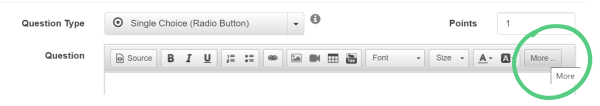5. Select the Math symbol.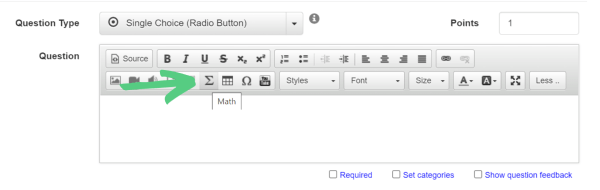6. A new window opens to enter the equation part of your question.
7. Enter your TeX Syntax code.
8. Press Ok
9. Your code will save as a professional-looking equation.
10. For example, when I enter the following TeX Syntax: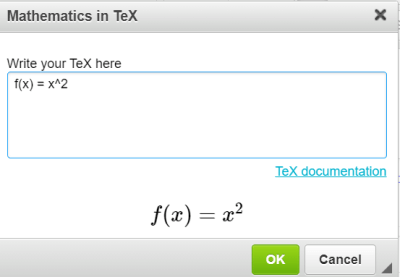11. This image shows how the math formulae looks to test-takers: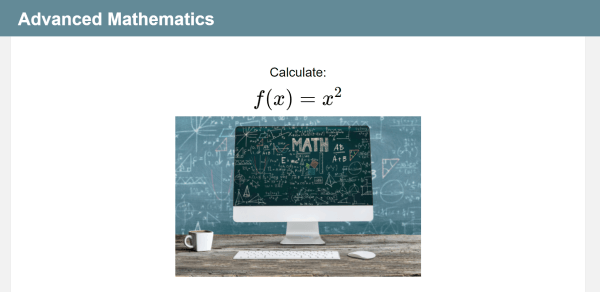12. To change the size of your formulae, hover over and choose a size from the drop-down menu.
13. Top tip! If you change the size of the text on the Theme screen, it will not change the size of the equation.
14. To view how the question looks to test-takers, press preview on the top right.
15. You can continue to add additional text, images, video, or audio as part of your question.
16. Finish configuring the test, publish, and share.

## How to add mathematical formulae and symbols to an answer option

2. Choose a relevant question type. You can add math formulae to the following question types:
3. Go to the answer options box and press the > arrow.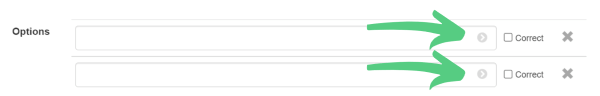4. Choose the Math symbol from the menu.
5. A new window opens for you to enter the mathematical answer option.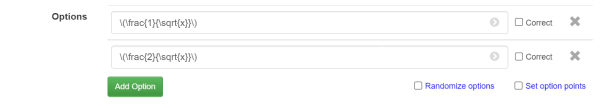6. Enter your Tex code and press ok
7. You can add additional text before or after the math formulae.
8. Save the question.